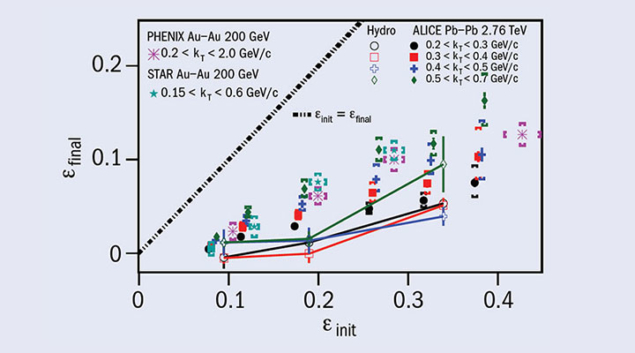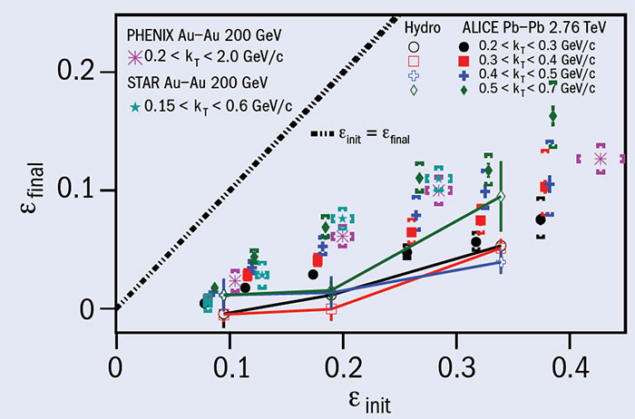# ALICE measures shape of the QGP fireball at freeze-out

17 March 2017Freeze-out eccentricity measured for different ranges of pair transverse momentum as a function of initial-state eccentricity obtained from Monte Carlo models for six centrality ranges, 0–5%, 5–10%, 10–20%, 20–30 %, 30–40 % and 40–50%.

Heavy-ion collisions at LHC energies create a hot and dense medium of deconfined quarks and gluons, known as the quark–gluon plasma (QGP). The QGP fireball first expands, cools and then freezes out into a collection of final-state hadrons. Correlations between the free particles carry information about the space–time extent of the emitting source, and are imprinted on the final-state spectra due to a quantum-mechanical interference effect. To measure these correlations and to determine the space–time parameters of the source, physicists utilise Hanbury Brown and Twiss (HBT) interferometry, a technique first used in astronomy for determining the angular sizes of stars. Using azimuthally differential HBT interferometry, the ALICE collaboration has recently measured the shape of the fireball at freeze-out.In a non-central collision, the nuclear overlap region is almond shaped with the longer axis oriented perpendicular to the reaction plane (defined by the impact parameter and the beam direction). The spatial anisotropies in the initial state are converted, via pressure gradients, to momentum anisotropies, leading to anisotropic particle flow. The magnitudes of the momentum anisotropies are quantified by the so-called vn coefficients, where the second harmonic coefficient (v2) is generated from the system’s approximately elliptic shape. This is usually called elliptic flow, and the direction of the strongest component of elliptic flow is defined as the elliptic-flow plane.

The HBT radius, measured as a function of the pair-emission azimuth relative to the elliptic-flow plane, exhibits oscillations and thus provides information on the eccentricity of the source at freeze-out, when the particles cease to interact. The source eccentricity at freeze-out can be estimated from oscillations of the HBT radius at low pion-pair transverse momentum. ALICE has measured the pion HBT-radius oscillations for different transverse-momentum ranges as a function of centrality in lead–lead collisions at an energy of 2.76 TeV per nucleon pair and plotted the results as a function of the initial eccentricity (see figure on previous page).

The final eccentricities are significantly below the initial eccentricities due to a larger expansion in the in-plane direction. The freeze-out eccentricities measured by ALICE are smaller than those measured at RHIC energies, likely reflecting the longer lifetime of the system at the LHC. Hydrodynamic calculations performed for similar centralities and pair transverse-momentum ranges as in the ALICE experiment show a similar trend, but predict smaller final-source eccentricity corresponding to a more spherical source.

The final-state source eccentricity remains positive for all the pair transverse-momentum ranges, indicating that even after a stronger expansion in the in-plane direction, the pion source at freeze-out is still elongated in the out-of-plane direction. In the future, the ALICE collaboration intends to measure the azimuthal dependence of the HBT radii relative to the higher-harmonic (n ≥ 3) flow planes, which is directly sensitive to anisotropies in the system’s collective velocity fields.